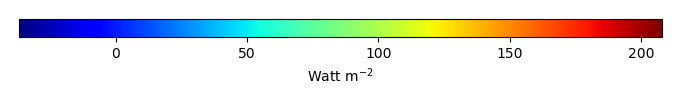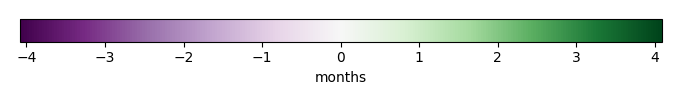# Mean State

Period Mean (original grids) [Watt m-2]
Model Period Mean (intersection) [Watt m-2]
Model Period Mean (complement) [Watt m-2]
Benchmark Period Mean (intersection) [Watt m-2]
Benchmark Period Mean (complement) [Watt m-2]
Bias [Watt m-2]
RMSE [Watt m-2]
Phase Shift [months]
Bias Score 
RMSE Score 
Seasonal Cycle Score 
Spatial Distribution Score 
Interannual Variability Score 
Overall Score 
Benchmark [-] 122.
CLM5PHSOFF [-] 116. 116. 120. 164. -4.34 18.2 1.11 0.671 0.435 0.852 0.908 0.690 0.665
CLM5PHSON [-] 116. 116. 120. 164. -3.73 17.7 0.987 0.682 0.444 0.868 0.893 0.670 0.667
Period Mean (original grids) [Watt m-2]
Model Period Mean (intersection) [Watt m-2]
Model Period Mean (complement) [Watt m-2]
Benchmark Period Mean (intersection) [Watt m-2]
Benchmark Period Mean (complement) [Watt m-2]
Bias [Watt m-2]
RMSE [Watt m-2]
Phase Shift [months]
Bias Score 
RMSE Score 
Seasonal Cycle Score 
Spatial Distribution Score 
Interannual Variability Score 
Overall Score 
Benchmark [-] 102.
CLM5PHSOFF [-] 96.4 95.9 101. 165. -4.73 20.0 1.24 0.655 0.514 0.814 0.865 0.694 0.676
CLM5PHSON [-] 97.7 97.2 101. 165. -3.30 19.8 1.28 0.668 0.513 0.808 0.890 0.651 0.674
Period Mean (original grids) [Watt m-2]
Model Period Mean (intersection) [Watt m-2]
Model Period Mean (complement) [Watt m-2]
Benchmark Period Mean (intersection) [Watt m-2]
Benchmark Period Mean (complement) [Watt m-2]
Bias [Watt m-2]
RMSE [Watt m-2]
Phase Shift [months]
Bias Score 
RMSE Score 
Seasonal Cycle Score 
Spatial Distribution Score 
Interannual Variability Score 
Overall Score 
Benchmark [-] 112.
CLM5PHSOFF [-] 104. 103. 110. 159. -6.56 21.3 0.873 0.677 0.567 0.884 0.846 0.654 0.699
CLM5PHSON [-] 105. 105. 110. 159. -3.73 20.8 1.22 0.712 0.566 0.815 0.873 0.615 0.691
Period Mean (original grids) [Watt m-2]
Model Period Mean (intersection) [Watt m-2]
Model Period Mean (complement) [Watt m-2]
Benchmark Period Mean (intersection) [Watt m-2]
Benchmark Period Mean (complement) [Watt m-2]
Bias [Watt m-2]
RMSE [Watt m-2]
Phase Shift [months]
Bias Score 
RMSE Score 
Seasonal Cycle Score 
Spatial Distribution Score 
Interannual Variability Score 
Overall Score 
Benchmark [-] 43.3
CLM5PHSOFF [-] 28.5 28.7 43.2 44.3 -14.5 25.8 0.239 0.772 0.683 0.984 0.789 0.757 0.778
CLM5PHSON [-] 33.3 33.3 43.2 44.3 -11.6 23.4 0.172 0.812 0.698 0.989 0.777 0.762 0.789
Period Mean (original grids) [Watt m-2]
Model Period Mean (intersection) [Watt m-2]
Model Period Mean (complement) [Watt m-2]
Benchmark Period Mean (intersection) [Watt m-2]
Benchmark Period Mean (complement) [Watt m-2]
Bias [Watt m-2]
RMSE [Watt m-2]
Phase Shift [months]
Bias Score 
RMSE Score 
Seasonal Cycle Score 
Spatial Distribution Score 
Interannual Variability Score 
Overall Score 
Benchmark [-] 144.
CLM5PHSOFF [-] 133. 132. 140. 160. -7.53 20.6 1.07 0.598 0.425 0.844 0.894 0.611 0.633
CLM5PHSON [-] 128. 127. 140. 160. -7.88 20.6 0.968 0.595 0.427 0.860 0.898 0.604 0.635
Period Mean (original grids) [Watt m-2]
Model Period Mean (intersection) [Watt m-2]
Model Period Mean (complement) [Watt m-2]
Benchmark Period Mean (intersection) [Watt m-2]
Benchmark Period Mean (complement) [Watt m-2]
Bias [Watt m-2]
RMSE [Watt m-2]
Phase Shift [months]
Bias Score 
RMSE Score 
Seasonal Cycle Score 
Spatial Distribution Score 
Interannual Variability Score 
Overall Score 
Benchmark [-] 62.5
CLM5PHSOFF [-] 42.8 42.7 62.3 75.7 -19.6 28.1 0.105 0.710 0.703 0.993 0.955 0.663 0.788
CLM5PHSON [-] 48.1 48.1 62.3 75.7 -13.9 23.3 0.107 0.785 0.722 0.993 0.935 0.666 0.804
Period Mean (original grids) [Watt m-2]
Model Period Mean (intersection) [Watt m-2]
Model Period Mean (complement) [Watt m-2]
Benchmark Period Mean (intersection) [Watt m-2]
Benchmark Period Mean (complement) [Watt m-2]
Bias [Watt m-2]
RMSE [Watt m-2]
Phase Shift [months]
Bias Score 
RMSE Score 
Seasonal Cycle Score 
Spatial Distribution Score 
Interannual Variability Score 
Overall Score 
Benchmark [-] 72.7
CLM5PHSOFF [-] 62.2 62.0 72.1 94.9 -10.1 22.2 0.376 0.768 0.652 0.971 0.992 0.626 0.777
CLM5PHSON [-] 62.7 62.6 72.1 94.9 -8.19 21.7 0.393 0.791 0.649 0.970 0.991 0.633 0.780
Period Mean (original grids) [Watt m-2]
Model Period Mean (intersection) [Watt m-2]
Model Period Mean (complement) [Watt m-2]
Benchmark Period Mean (intersection) [Watt m-2]
Benchmark Period Mean (complement) [Watt m-2]
Bias [Watt m-2]
RMSE [Watt m-2]
Phase Shift [months]
Bias Score 
RMSE Score 
Seasonal Cycle Score 
Spatial Distribution Score 
Interannual Variability Score 
Overall Score 
Benchmark [-] 109.
CLM5PHSOFF [-] 96.5 95.7 105. 157. -9.55 24.3 0.467 0.677 0.536 0.957 0.959 0.572 0.706
CLM5PHSON [-] 94.4 94.1 105. 157. -6.92 23.7 0.544 0.716 0.528 0.952 0.958 0.560 0.707
Period Mean (original grids) [Watt m-2]
Model Period Mean (intersection) [Watt m-2]
Model Period Mean (complement) [Watt m-2]
Benchmark Period Mean (intersection) [Watt m-2]
Benchmark Period Mean (complement) [Watt m-2]
Bias [Watt m-2]
RMSE [Watt m-2]
Phase Shift [months]
Bias Score 
RMSE Score 
Seasonal Cycle Score 
Spatial Distribution Score 
Interannual Variability Score 
Overall Score 
Benchmark [-] 41.8
CLM5PHSOFF [-] 22.6 23.0 41.9 41.5 -18.8 24.3 0.236 0.707 0.693 0.984 0.948 0.748 0.796
CLM5PHSON [-] 25.1 25.4 41.9 41.5 -17.5 23.3 0.253 0.725 0.696 0.983 0.940 0.755 0.799
Period Mean (original grids) [Watt m-2]
Model Period Mean (intersection) [Watt m-2]
Model Period Mean (complement) [Watt m-2]
Benchmark Period Mean (intersection) [Watt m-2]
Benchmark Period Mean (complement) [Watt m-2]
Bias [Watt m-2]
RMSE [Watt m-2]
Phase Shift [months]
Bias Score 
RMSE Score 
Seasonal Cycle Score 
Spatial Distribution Score 
Interannual Variability Score 
Overall Score 
Benchmark [-] 96.5
CLM5PHSOFF [-] 92.9 92.8 93.2 138. -0.576 20.1 0.376 0.777 0.668 0.971 0.847 0.661 0.765
CLM5PHSON [-] 92.5 92.4 93.2 138. 1.15 20.2 0.330 0.778 0.666 0.974 0.866 0.637 0.764
Period Mean (original grids) [Watt m-2]
Model Period Mean (intersection) [Watt m-2]
Model Period Mean (complement) [Watt m-2]
Benchmark Period Mean (intersection) [Watt m-2]
Benchmark Period Mean (complement) [Watt m-2]
Bias [Watt m-2]
RMSE [Watt m-2]
Phase Shift [months]
Bias Score 
RMSE Score 
Seasonal Cycle Score 
Spatial Distribution Score 
Interannual Variability Score 
Overall Score 
Benchmark [-] 64.4
CLM5PHSOFF [-] 54.5 54.4 61.9 90.5 -7.54 19.0 0.276 0.846 0.749 0.982 0.989 0.634 0.825
CLM5PHSON [-] 54.4 54.4 61.9 90.5 -5.49 19.0 0.289 0.858 0.742 0.981 0.985 0.640 0.825
Period Mean (original grids) [Watt m-2]
Model Period Mean (intersection) [Watt m-2]
Model Period Mean (complement) [Watt m-2]
Benchmark Period Mean (intersection) [Watt m-2]
Benchmark Period Mean (complement) [Watt m-2]
Bias [Watt m-2]
RMSE [Watt m-2]
Phase Shift [months]
Bias Score 
RMSE Score 
Seasonal Cycle Score 
Spatial Distribution Score 
Interannual Variability Score 
Overall Score 
Benchmark [-] 115.
CLM5PHSOFF [-] 65.5 65.5 77.4 133. -12.0 24.6 0.547 0.660 0.595 0.937 0.926 0.630 0.724
CLM5PHSON [-] 65.7 65.7 77.4 133. -10.1 23.7 0.563 0.682 0.597 0.933 0.922 0.623 0.726
Period Mean (original grids) [Watt m-2]
Model Period Mean (intersection) [Watt m-2]
Model Period Mean (complement) [Watt m-2]
Benchmark Period Mean (intersection) [Watt m-2]
Benchmark Period Mean (complement) [Watt m-2]
Bias [Watt m-2]
RMSE [Watt m-2]
Phase Shift [months]
Bias Score 
RMSE Score 
Seasonal Cycle Score 
Spatial Distribution Score 
Interannual Variability Score 
Overall Score 
Benchmark [-] 54.9
CLM5PHSOFF [-] 55.7 55.6 51.3 143. 4.35 25.9 0.893 0.670 0.519 0.888 0.837 0.548 0.664
CLM5PHSON [-] 54.2 54.2 51.3 143. 5.15 26.1 0.880 0.664 0.516 0.890 0.852 0.552 0.665
Period Mean (original grids) [Watt m-2]
Model Period Mean (intersection) [Watt m-2]
Model Period Mean (complement) [Watt m-2]
Benchmark Period Mean (intersection) [Watt m-2]
Benchmark Period Mean (complement) [Watt m-2]
Bias [Watt m-2]
RMSE [Watt m-2]
Phase Shift [months]
Bias Score 
RMSE Score 
Seasonal Cycle Score 
Spatial Distribution Score 
Interannual Variability Score 
Overall Score 
Benchmark [-] 44.9
CLM5PHSOFF [-] 24.9 24.8 44.8 45.6 -20.0 25.9 0.133 0.700 0.713 0.991 0.902 0.776 0.799
CLM5PHSON [-] 28.4 28.3 44.8 45.6 -18.6 24.6 0.122 0.720 0.720 0.992 0.875 0.780 0.801
Period Mean (original grids) [Watt m-2]
Model Period Mean (intersection) [Watt m-2]
Model Period Mean (complement) [Watt m-2]
Benchmark Period Mean (intersection) [Watt m-2]
Benchmark Period Mean (complement) [Watt m-2]
Bias [Watt m-2]
RMSE [Watt m-2]
Phase Shift [months]
Bias Score 
RMSE Score 
Seasonal Cycle Score 
Spatial Distribution Score 
Interannual Variability Score 
Overall Score 
Benchmark [-] 134.
CLM5PHSOFF [-] 110. 111. 127. 169. -16.2 25.3 0.736 0.613 0.603 0.913 0.945 0.639 0.719
CLM5PHSON [-] 107. 108. 127. 169. -14.0 24.1 0.799 0.639 0.605 0.898 0.949 0.624 0.720
Period Mean (original grids) [Watt m-2]
Model Period Mean (intersection) [Watt m-2]
Model Period Mean (complement) [Watt m-2]
Benchmark Period Mean (intersection) [Watt m-2]
Benchmark Period Mean (complement) [Watt m-2]
Bias [Watt m-2]
RMSE [Watt m-2]
Phase Shift [months]
Bias Score 
RMSE Score 
Seasonal Cycle Score 
Spatial Distribution Score 
Interannual Variability Score 
Overall Score 
Benchmark [-] 99.6
CLM5PHSOFF [-] 80.3 80.2 97.8 136. -17.6 24.1 0.345 0.701 0.721 0.974 0.992 0.629 0.790
CLM5PHSON [-] 82.0 82.1 97.8 136. -14.0 21.5 0.352 0.754 0.723 0.974 0.998 0.625 0.800
Period Mean (original grids) [Watt m-2]
Model Period Mean (intersection) [Watt m-2]
Model Period Mean (complement) [Watt m-2]
Benchmark Period Mean (intersection) [Watt m-2]
Benchmark Period Mean (complement) [Watt m-2]
Bias [Watt m-2]
RMSE [Watt m-2]
Phase Shift [months]
Bias Score 
RMSE Score 
Seasonal Cycle Score 
Spatial Distribution Score 
Interannual Variability Score 
Overall Score 
Benchmark [-] 72.2
CLM5PHSOFF [-] 53.5 53.7 72.0 77.2 -18.4 25.3 0.109 0.726 0.740 0.993 0.994 0.674 0.811
CLM5PHSON [-] 58.6 58.7 72.0 77.2 -13.2 20.6 0.102 0.792 0.759 0.993 0.996 0.667 0.828
Period Mean (original grids) [Watt m-2]
Model Period Mean (intersection) [Watt m-2]
Model Period Mean (complement) [Watt m-2]
Benchmark Period Mean (intersection) [Watt m-2]
Benchmark Period Mean (complement) [Watt m-2]
Bias [Watt m-2]
RMSE [Watt m-2]
Phase Shift [months]
Bias Score 
RMSE Score 
Seasonal Cycle Score 
Spatial Distribution Score 
Interannual Variability Score 
Overall Score 
Benchmark [-] 81.6
CLM5PHSOFF [-] 69.1 69.4 81.1 116. -11.6 22.0 0.312 0.756 0.708 0.977 0.993 0.659 0.800
CLM5PHSON [-] 72.0 72.2 81.1 116. -8.22 20.4 0.314 0.793 0.706 0.977 0.994 0.660 0.806
Period Mean (original grids) [Watt m-2]
Model Period Mean (intersection) [Watt m-2]
Model Period Mean (complement) [Watt m-2]
Benchmark Period Mean (intersection) [Watt m-2]
Benchmark Period Mean (complement) [Watt m-2]
Bias [Watt m-2]
RMSE [Watt m-2]
Phase Shift [months]
Bias Score 
RMSE Score 
Seasonal Cycle Score 
Spatial Distribution Score 
Interannual Variability Score 
Overall Score 
Benchmark [-] 132.
CLM5PHSOFF [-] 122. 122. 131. 178. -8.95 21.0 0.945 0.601 0.392 0.866 0.959 0.617 0.638
CLM5PHSON [-] 122. 122. 131. 178. -7.96 20.3 0.991 0.616 0.395 0.859 0.949 0.603 0.636
Period Mean (original grids) [Watt m-2]
Model Period Mean (intersection) [Watt m-2]
Model Period Mean (complement) [Watt m-2]
Benchmark Period Mean (intersection) [Watt m-2]
Benchmark Period Mean (complement) [Watt m-2]
Bias [Watt m-2]
RMSE [Watt m-2]
Phase Shift [months]
Bias Score 
RMSE Score 
Seasonal Cycle Score 
Spatial Distribution Score 
Interannual Variability Score 
Overall Score 
Benchmark [-] 117.
CLM5PHSOFF [-] 106. 106. 117. 145. -10.9 21.6 0.959 0.681 0.554 0.873 0.980 0.646 0.715
CLM5PHSON [-] 108. 108. 117. 145. -8.04 20.3 0.874 0.730 0.559 0.885 0.968 0.621 0.720
Period Mean (original grids) [Watt m-2]
Model Period Mean (intersection) [Watt m-2]
Model Period Mean (complement) [Watt m-2]
Benchmark Period Mean (intersection) [Watt m-2]
Benchmark Period Mean (complement) [Watt m-2]
Bias [Watt m-2]
RMSE [Watt m-2]
Phase Shift [months]
Bias Score 
RMSE Score 
Seasonal Cycle Score 
Spatial Distribution Score 
Interannual Variability Score 
Overall Score 
Benchmark [-] 95.7
CLM5PHSOFF [-] 88.6 87.8 92.5 124. -4.73 25.3 0.388 0.728 0.681 0.964 0.987 0.565 0.768
CLM5PHSON [-] 90.8 90.1 92.5 124. -3.40 24.9 0.391 0.740 0.681 0.963 0.988 0.568 0.770

# Temporally integrated period mean

BENCHMARK MEANMODEL MEANBIASBIAS SCORERMSERMSE SCOREBENCHMARK INTERANNUAL VARIABILITYMODEL INTERANNUAL VARIABILITYINTERANNUAL VARIABILITY SCOREBENCHMARK MAX MONTHMODEL MAX MONTHDIFFERENCE IN MAX MONTHSEASONAL CYCLE SCORESPATIAL TAYLOR DIAGRAMMODEL COLORS# Spatially integrated regional mean

MODEL COLORSREGIONAL MEANANNUAL CYCLEMONTHLY ANOMALYANNUAL CYCLE# All Models

BenchmarkCLM5PHSOFFCLM5PHSON# Data Information

creation_date: Tue Jul 1 08:16:58 PDT 2014

Conventions: Please contact Prof. James Randerson (Email: jranders@uci.edu) or Dr. Mingquan Mu (mmu@uci.edu) for any question

source_file: This product is generated from monthly 1 degree GEWEX SRB Radiation observations

title: derived GEWEX SRB Radiation

Approach: To obtain surface net radiation from GEWEX SRB radiation data, first I subtracted surface all-sky upward shortwave (sw_sfc_up) from surface all-sky downward shortwave (sw_sfc_dn) to get surface net shortwave radiation(rsns). Using the same way, I also got surface net longwave radiation (rlns = lw_sfc_dn-lw_sfc_up). Then, I added rsns and rlns to get surface net radiation (rns). Meanwhile, the data were also binned from original 1.0x1.0 resolution to final 0.5x0.5 resolution.

Temporal resolution: monthly

General information: This product was derived from NASA GEWEX SRB radiation dataset.

Spatial resolution: 0.5x0.5 degree